## The first law of thermodynamics

12-8-99

Sections 15.1 - 15.4

### Thermodynamics

Thermodynamics is the study of systems involving energy in the form of heat and work. A good example of a thermodynamic system is gas confined by a piston in a cylinder. If the gas is heated, it will expand, doing work on the piston; this is one example of how a thermodynamic system can do work.

Thermal equilibrium is an important concept in thermodynamics. When two systems are in thermal equilibrium, there is no net heat transfer between them. This occurs when the systems are at the same temperature. In other words, systems at the same temperature will be in thermal equilibrium with each other.

The first law of thermodynamics relates changes in internal energy to heat added to a system and the work done by a system. The first law is simply a conservation of energy equation: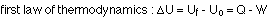The internal energy has the symbol U. Q is positive if heat is added to the system, and negative if heat is removed; W is positive if work is done by the system, and negative if work is done on the system.

We've talked about how heat can be transferred, so you probably have a good idea about what Q means in the first law. What does it mean for the system to do work? Work is simply a force multiplied by the distance moved in the direction of the force. A good example of a thermodynamic system that can do work is the gas confined by a piston in a cylinder, as shown in the diagram.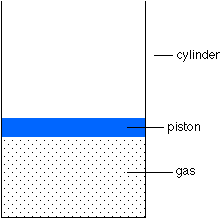If the gas is heated, it will expand and push the piston up, thereby doing work on the piston. If the piston is pushed down, on the other hand, the piston does work on the gas and the gas does negative work on the piston. This is an example of how work is done by a thermodynamic system. An example with numbers might make this clearer.

### An example of work done

Consider a gas in a cylinder at room temperature (T = 293 K), with a volume of 0.065 m3. The gas is confined by a piston with a weight of 100 N and an area of 0.65 m2. The pressure above the piston is atmospheric pressure.

(a) What is the pressure of the gas?

This can be determined from a free-body diagram of the piston. The weight of the piston acts down, and the atmosphere exerts a downward force as well, coming from force = pressure x area. These two forces are balanced by the upward force coming from the gas pressure. The piston is in equilibrium, so the forces balance. Therefore: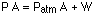Solving for the pressure of the gas gives: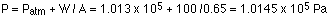The pressure in the gas isn't much bigger than atmospheric pressure, just enough to support the weight of the piston.

(b) The gas is heated, expanding it and moving the piston up. If the volume occupied by the gas doubles, how much work has the gas done?

An assumption to make here is that the pressure is constant. Once the gas has expanded, the pressure will certainly be the same as before because the same free-body diagram applies. As long as the expansion takes place slowly, it is reasonable to assume that the pressure is constant.

If the volume has doubled, then, and the pressure has remained the same, the ideal gas law tells us that the temperature must have doubled too.

The work done by the gas can be determined by working out the force applied by the gas and calculating the distance. However, the force applied by the gas is the pressure times the area, so:

W = F s = P A s

and the area multiplied by the distance is a volume, specifically the change in volume of the gas. So, at constant pressure, work is just the pressure multiplied by the change in volume:This is positive because the force and the distance moved are in the same direction, so this is work done by the gas.

### The pressure-volume graph

As has been discussed, a gas enclosed by a piston in a cylinder can do work on the piston, the work being the pressure multiplied by the change in volume. If the volume doesn't change, no work is done. If the pressure stays constant while the volume changes, the work done is easy to calculate. On the other hand, if pressure and volume are both changing it's somewhat harder to calculate the work done.

As an aid in calculating the work done, it's a good idea to draw a pressure-volume graph (with pressure on the y axis and volume on the x-axis). If a system moves from one point on the graph to another and a line is drawn to connect the points, the work done is the area underneath this line. We'll go through some different thermodynamic processes and see how this works.

### Types of thermodynamic processes

There are a number of different thermodynamic processes that can change the pressure and/or the volume and/or the temperature of a system. To simplify matters, consider what happens when something is kept constant. The different processes are then categorized as follows :

1. Isobaric - the pressure is kept constant. An example of an isobaric system is a gas, being slowly heated or cooled, confined by a piston in a cylinder. The work done by the system in an isobaric process is simply the pressure multiplied by the change in volume, and the P-V graph looks like:2. Isochoric - the volume is kept constant. An example of this system is a gas in a box with fixed walls. The work done is zero in an isochoric process, and the P-V graph looks like:3. Isothermal - the temperature is kept constant. A gas confined by a piston in a cylinder is again an example of this, only this time the gas is not heated or cooled, but the piston is slowly moved so that the gas expands or is compressed. The temperature is maintained at a constant value by putting the system in contact with a constant-temperature reservoir (the thermodynamic definition of a reservoir is something large enough that it can transfer heat into or out of a system without changing temperature).

If the volume increases while the temperature is constant, the pressure must decrease, and if the volume decreases the pressure must increase.

The isothermal and adiabatic processes should be examined in a little more detail.

### Isothermal processes

In an isothermal process, the temperature stays constant, so the pressure and volume are inversely proportional to one another. The P-V graph for an isothermal process looks like this: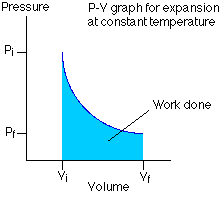The work done by the system is still the area under the P-V curve, but because this is not a straight line the calculation is a little tricky, and really can only properly be done using calculus.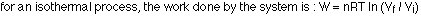The internal energy of an ideal gas is proportional to the temperature, so if the temperature is kept fixed the internal energy does not change. The first law, which deals with changes in the internal energy, thus becomes 0 = Q - W, so Q = W. If the system does work, the energy comes from heat flowing into the system from the reservoir; if work is done on the system, heat flows out of the system to the reservoir.

In an adiabatic process, no heat is added or removed from a system. The first law of thermodynamics is thus reduced to saying that the change in the internal energy of a system undergoing an adiabatic change is equal to -W. Since the internal energy is directly proportional to temperature, the work becomes: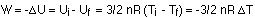An example of an adiabatic process is a gas expanding so quickly that no heat can be transferred. The expansion does work, and the temperature drops. This is exactly what happens with a carbon dioxide fire extinguisher, with the gas coming out at high pressure and cooling as it expands at atmospheric pressure.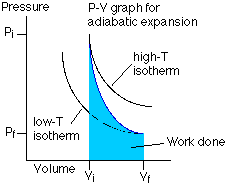### Specific heat capacity of an ideal gas

With liquids and solids that are changing temperature, the heat associated with a temperature change is given by the equation:A similar equation holds for an ideal gas, only instead of writing the equation in terms of the mass of the gas it is written in terms of the number of moles of gas, and use a capital C for the heat capacity, with units of J / (mol K):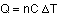For an ideal gas, the heat capacity depends on what kind of thermodynamic process the gas is experiencing. Generally, two different heat capacities are stated for a gas, the heat capacity at constant pressure (Cp) and the heat capacity at constant volume (Cv). The value at constant pressure is larger than the value at constant volume because at constant pressure not all of the heat goes into changing the temperature; some goes into doing work. On the other hand, at constant volume no work is done, so all the heat goes into changing the temperature. In other words, it takes less heat to produce a given temperature change at constant volume than it does at constant pressure, so Cv < Cp.

That's a qualitative statement about the two different heat capacities, but it's very easy to examine them quantitatively. The first law says:We also know that PV = nRT, and at constant pressure the work done is: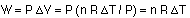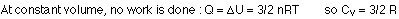Note that this applies for a monatomic ideal gas. For all gases, though, the following is true: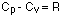Another important number is the ratio of the two specific heats, represented by the Greek letter gamma (g). For a monatomic ideal gas this ratio is: